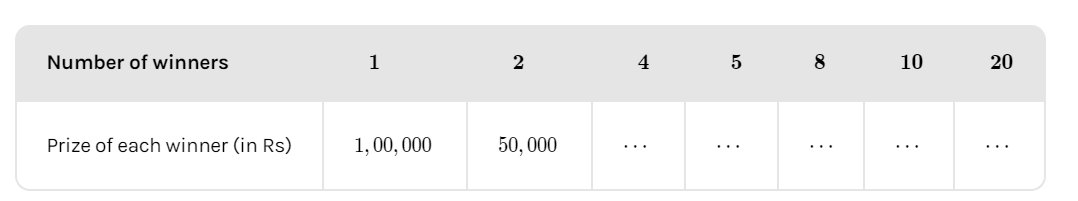QuestionAnswers

# In a television game show, the prize money of Rs.1, 00,000 is to be divided equally amongst the winners. Complete the following table and find whether the prize money given to an individual winner is directly or inversely proportional to the number of winners?Hint: Here, we will find the relation between the prize money of an individual
winner and the number of winners in a game.

Given,
Prize money of the game show is 1, 00,000. Let us consider ‘x’ be the number of winners and
‘y’ be the value of prize for each winner. Therefore, we can consider the following equation

$x*y = 100000 \to (1)$
Also given, that the prize money for a single winner is 1, 00,000 and for ‘2’ winners is 50,000.Now we need to find the prize money if the number of winners are 4, 5,8,10 and 20.
To do so we need to substitute the number of prize winners in ‘x’ and find the value of ‘y’ variable i.e.., prize of each winner.
For $x = 4$, we get $4*y = 1,00,000 \Rightarrow y = \frac{{100000}}{4} = 25,000$.
Hence, the amount won by each winner will be 25,000 if the number of winners in the game
show are 4.
Similarly, let us substitute the values of 5,8,10 and 20 as well, we get
$x = 5 \Rightarrow 5*y = 1,00,000 \Rightarrow y = \frac{{100000}}{5} = 20,000 \\ x = 8 \Rightarrow 8*y = 1,00,000 \Rightarrow y = \frac{{100000}}{8} = 12,500 \\ x = 10 \Rightarrow 10*y = 1,00,000 \Rightarrow y = \frac{{100000}}{{10}} = 10,000 \\ x = 20 \Rightarrow 20*y = 1,00,000 \Rightarrow y = \frac{{100000}}{{20}} = 5,000 \\$
Let us complete the given table with the obtained values. We get

 Number of winners $1$ $2$ $4$ $5$ $8$ $10$ $20$ Prize of each winner (in Rs) $1,00,000$ $50,000$ $25,000$ $20,000$ $12,500$ $10,000$ $5,000$

As we can see the number of winners in the game show are increasing and the prize money
of each winner is decreasing. Hence, we can make a conclusion that the prize money given
to an individual winner is inversely proportional to the number of winners.
Prize money of an individual winner $\propto \frac{1}{{{\text{number of winners}}}}$.

Note: Since the total amount of prize money is the same for the game show irrespective of
number of winners, we have used the concept of linear equations and concluded the
relation between the prize money and number of winners as “inverse proportion”.
View Notes
Game TheoryTelevision EssayDirect and Inverse ProportionSupply of MoneyFunctions of MoneyDirect and Inverse Proportion MCQsQuantity Theory of MoneyMy Favourite Game EssayMoney MarketTable of 7